StatLect

# Discrete Fourier Transform - Frequencies

The Discrete Fourier Transform (DFT) is used to analyze the frequencies of a signal.

But what are these frequencies exactly?

Sometimes, the terminology can get a little bit confusing.

To check how the terms are used, we analyzed more than 50 different sources (books and lecture notes).## Aim

Our aim is to clearly explain the meaning of the following terms:

• sampling period (or interval, or time);

• sampling frequency (or rate);

• fundamental period (or total sampling time, or measurement period, or time gate);

• fundamental frequency (or frequency resolution, or frequency increment, or bin width);

• harmonic frequencies;

• frequency bins.

Moreover, we will discuss angular and normalized frequencies.

## Sampling of a continuous signal

We often use the Discrete Fourier Transform to analyze a continuous signal that has been sampled at discrete time intervals.

In other words, we can observe the signalat any time, but we record its value everyseconds: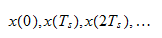## Sampling period

The time intervalbetween two samples is called sampling period. It is expressed in seconds.

For example,means that we take a sample every two seconds.

• Synonyms: sampling time; sampling interval.

• Alternative notation:.

## Sampling frequency

The sampling frequencyis the reciprocal of the sampling period:It is measured in hertz (Hz = 1 / s) or samples per second.

For example,means that we take two samples per second.

• Synonyms: sampling rate.

## Number of samples

The total number of samples is denoted by.

In other words, we record the samples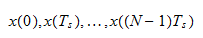## Fundamental period

The fundamental periodis defined asTherefore, the number of samples is equal to the product of the fundamental period and the sampling frequency:• Synonyms: total sampling time, measurement period, time gate.

• Alternative notation:.

## Remarks about the fundamental period

In general, the fundamental period of a periodic signalis the smallestsuch thatfor any.

In a DFT setting, the fundamental period (defined as number of samples times sampling interval) may not coincide with the fundamental period of the signal.

However, when the DFT is used to analyze the discretized signal, the latter is treated as a periodic function with fundamental period, even if the original signal had a different period.

## Fundamental frequency

The fundamental frequencyis the reciprocal of the fundamental period: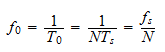• Synonyms: frequency resolution; frequency increment; bin width.

• Alternative notation:.

## Angular frequencies

Frequencies are sometimes expressed as angular frequencies, in radians per second (rad / s).

To get an angular frequency, we need to multiply a "regular" frequency by.

For example, if we use angular frequencies, the fundamental frequencyis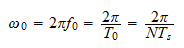and the sampling frequency is## Normalized frequencies

Frequencies can also be expressed as normalized frequencies, in cycles per sample.

Given a frequency, its normalized versionis obtained as:whereis the sampling rate.

For example, the normalized fundamental frequency isIn other words, a signal at the fundamental frequency completescycles per sample.

## The DFT and its inverse

Let us now look at the frequencies used in the DFT.

Thesamplesare denoted byFor, the Discrete Fourier Transform of the sampled signal is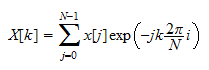whereis the imaginary unit.

For, the inverse DFT is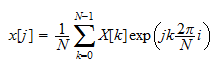In other words, the samples are linear combinations of thebasis functions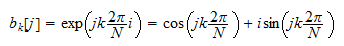for.

## Frequencies of the basis functions

The basis functioncompletes one full cycle insamples. Therefore, its period is equal to the fundamental period.

The basis functioncompletes one full cycle insamples.

As a consequence, the period ofis a fraction of the fundamental period:The frequency ofis the reciprocal of its period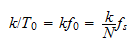whereis the fundamental frequency andis the sampling frequency.

In other words, the frequency ofistimes the fundamental frequency. It is also proportional to the sampling frequency.

The normalized frequency of(i.e., its frequency divided by the sampling frequency) is## Harmonic frequencies

We have seen above that the frequencies of the basis functions are integer multiples of the fundamental frequency: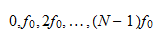These frequencies are known as harmonic frequencies.

The frequencyis the-th harmonic.

• Synonyms: harmonics.

## Frequency bins

The indicesof the basis functionsare often referred to as frequency bins.

However, for some authors the-th bin is a set of frequencies around the-th harmonic frequency.

• Synonyms: bin indices.

## DC bin

The-th bin in called DC bin and the other bins are called non-DC.

The corresponding frequencies are called DC frequencies and non-DC frequencies.

## Nyquist frequency

The Nyquist frequency isIt has a crucial role in the sampling theorem, which states that a continuous signal can be perfectly reconstructed from its samples taken at the sampling rateprovided that the signal contains only frequencies less than.

• Synonyms: folding frequency.